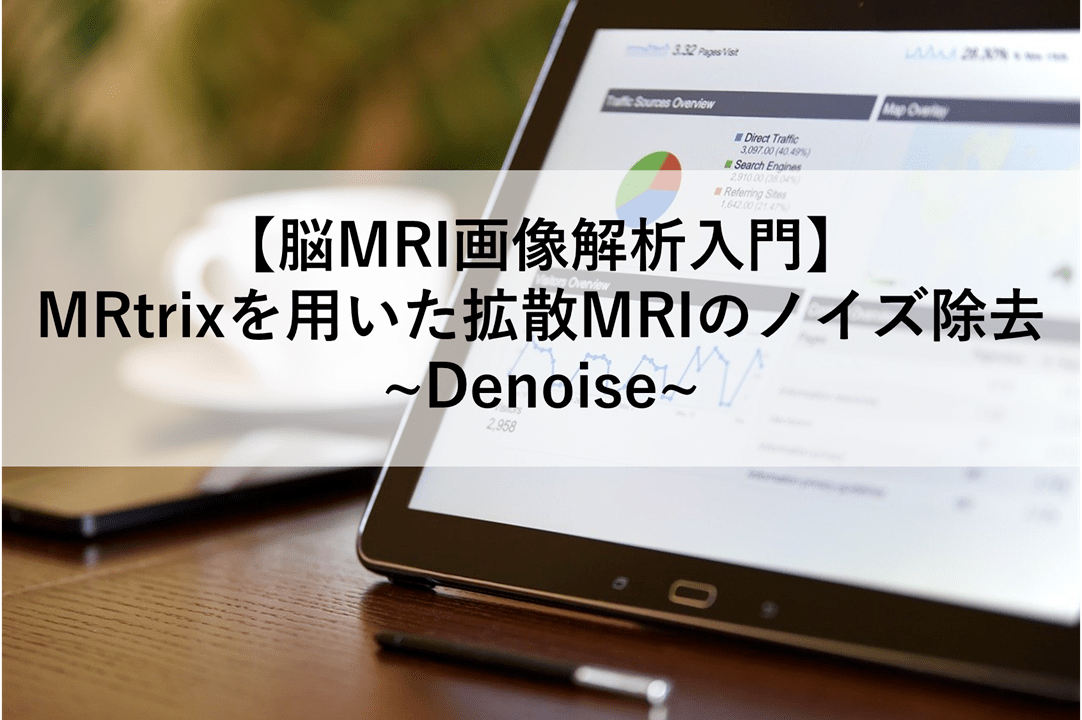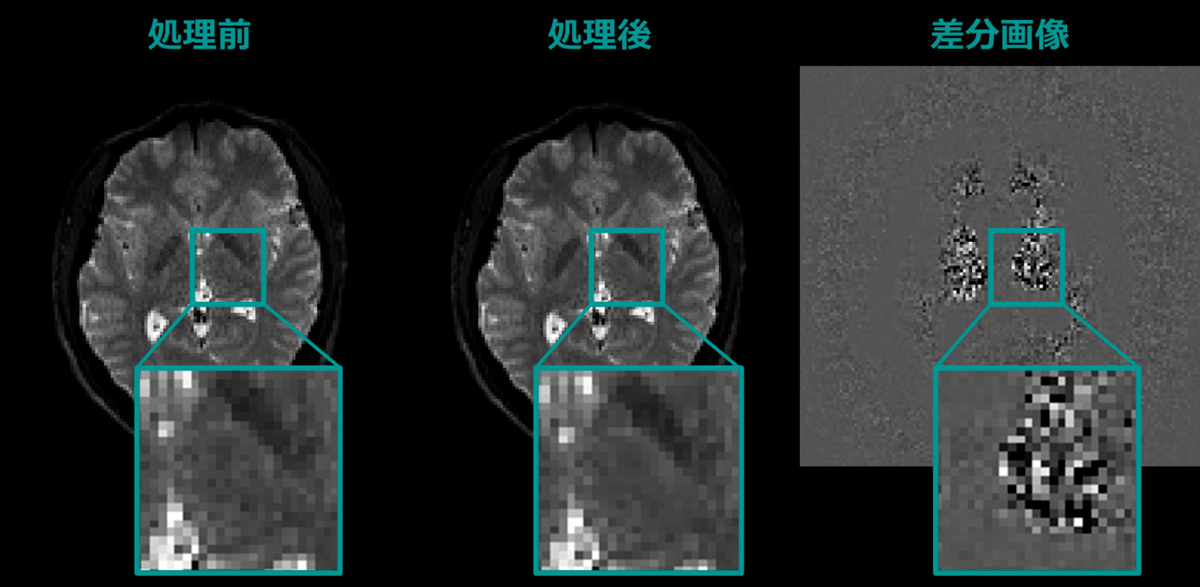# 【MRtrix】MRtrixを用いた拡散MRIのノイズ除去 ~Denoise~1. 目的
2. コマンド
2.1. 使用例

### 1. 目的

• MRtrixを用いた拡散MRIのノイズ除去（Denoise）

### 2. コマンド

`dwidenoise`のヘルプは、次の通り。

クリックして展開
```SYNOPSIS

dMRI noise level estimation and denoising using Marchenko-Pastur PCA

USAGE

dwidenoise [ options ] dwi out

dwi          the input diffusion-weighted image.

out          the output denoised DWI image.

DESCRIPTION

DWI data denoising and noise map estimation by exploiting data redundancy
in the PCA domain using the prior knowledge that the eigenspectrum of
random covariance matrices is described by the universal Marchenko-Pastur
(MP) distribution. Fitting the MP distribution to the spectrum of
patch-wise signal matrices hence provides an estimator of the noise level
'sigma', as was first shown in Veraart et al. (2016) and later improved in
Cordero-Grande et al. (2019). This noise level estimate then determines
the optimal cut-off for PCA denoising.

Important note: image denoising must be performed as the first step of the
image processing pipeline. The routine will fail if interpolation or
smoothing has been applied to the data prior to denoising.

Note that this function does not correct for non-Gaussian noise biases
present in magnitude-reconstructed MRI images. If available, including the
MRI phase data can reduce such non-Gaussian biases, and the command now
supports complex input data.

OPTIONS

Only process voxels within the specified binary brain mask image.

-extent window
Set the patch size of the denoising filter. By default, the command will
select the smallest isotropic patch size that exceeds the number of DW
images in the input data, e.g., 5x5x5 for data with <= 125 DWI volumes,
7x7x7 for data with <= 343 DWI volumes, etc.

-noise level
The output noise map, i.e., the estimated noise level 'sigma' in the data.
Note that on complex input data, this will be the total noise level across
real and imaginary channels, so a scale factor sqrt(2) applies.

-datatype float32/float64
Datatype for the eigenvalue decomposition (single or double precision).
For complex input data, this will select complex float32 or complex
float64 datatypes.

-estimator Exp1/Exp2
Select the noise level estimator (default = Exp2), either:
* Exp1: the original estimator used in Veraart et al. (2016), or
* Exp2: the improved estimator introduced in Cordero-Grande et al. (2019).

Standard options

-info
display information messages.

-quiet
do not display information messages or progress status; alternatively,
this can be achieved by setting the MRTRIX_QUIET environment variable to a
non-empty string.

-debug
display debugging messages.

-force
force overwrite of output files (caution: using the same file as input and
output might cause unexpected behaviour).

use this number of threads in multi-threaded applications (set to 0 to

-config key value  (multiple uses permitted)
temporarily set the value of an MRtrix config file entry.

-help
display this information page and exit.

-version
display version information and exit.
```

```dwidenoise <入力画像> <出力画像>
```

#### 2.1. 使用例

```dwidenoise DWI.nii.gz DWI_denoised.nii.gz
```このサイトはスパムを低減するために Akismet を使っています。コメントデータの処理方法の詳細はこちらをご覧ください